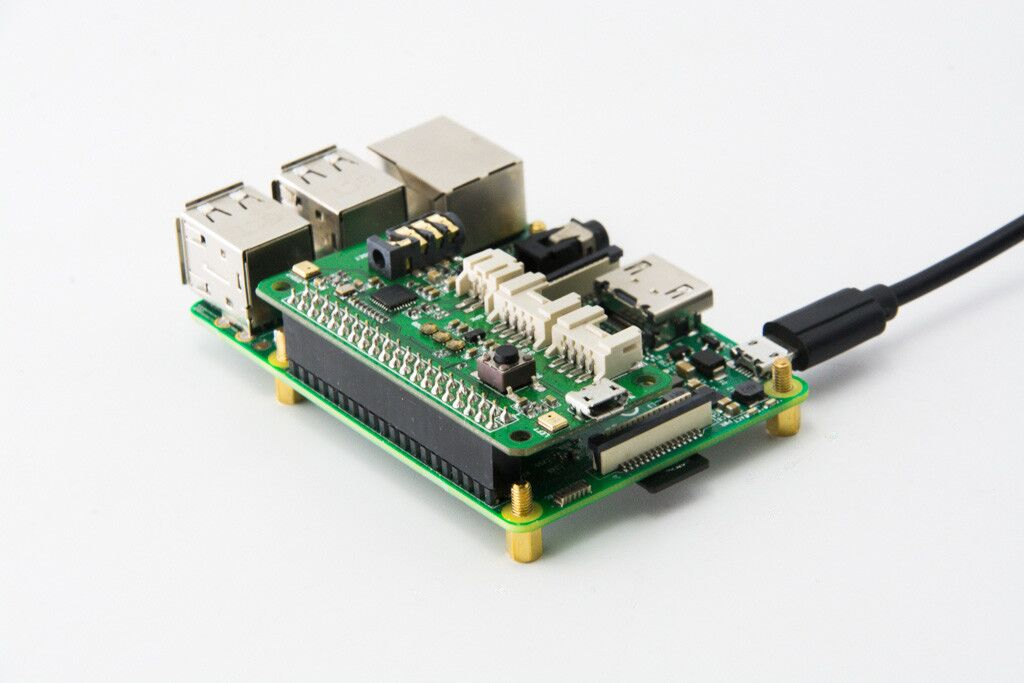Configuring LED Lights with SUSI Smart Speaker

To make the SUSI Smart Speaker more interactive and to improve the visual aesthetics, we configured SUSI Smart Speaker’s response with 3 RGB led lights. We have used a new piHat as an external hardware to configure the LEDs.

Now the new hardware specs of the SUSI Smart Speaker are:

1. Raspberry Pi
2. ReSpeaker PiHat 2 Mic Array
3. External Speakers

Using an external PiHat not only added the RGB light functionality but also eliminated the need to use a USB microphone and configured a factory reset button

Configuring the PiHat as the default Audio driver

To Use the PiHat as the default input driver, we use the package called PulseAudio.

And we use the following command in the installation script.

Configuring PiHat’s GPIO Button with Factory Reset

There is an onboard User Button, which is connected to GPIO17. We use the python library RPi.GPIO to detect the user button. The python script is used in the following way

 GPIO.setmode(GPIO.BCM) GPIO.setup(17,GPIO.IN) i = 1 while True: if GPIO.input(17) == 1:        time.sleep(0.1)        pass    elif GPIO.input(17) == 0 :        start = time.time()        while GPIO.input(17) == 0 :            time.sleep(0.1)        end = time.time()        total = end – start        if total >= 7 :            subprocess.call([‘bash’,‘factory_reset.sh’])  # nosec #pylint-disable type: ignore        else :            mixer = alsaaudio.Mixer()            value = mixer.getvolume()            if value != 0:                mixer.setvolume(0)            else:                mixer.setvolume(50)        print(total)        time.sleep(0.1)

This script checks on the button which is configured on GPIO port 17 on the PiHat. If the button is pressed for than 7 secs, the factory reset process takes place, else the device is muted.

Configuring PiHat’s LED with Speaker’s Response

We use a python library called SPIDEV to sync the LED lights with SUSI’s response. SPIDEV is usually used to send a response to the bus devices on the Raspberry Pi.

The first step was installing spidev

 sudo pip install spidev

Now we create a class where we store all the methods where we send the signal to the bus port. We treat the LED lights as a circular array and then have a rotation of RGB lights

 class LED_COLOR:     # Constants    MAX_BRIGHTNESS = 0b11111    LED_START = 0b11100000     def __init__(self, num_led, global_brightness=MAX_BRIGHTNESS,                 order=‘rgb’, bus=0, device=1, max_speed_hz=8000000):        self.num_led = num_led        order = order.lower()        self.rgb = RGB_MAP.get(order, RGB_MAP[‘rgb’])        if global_brightness > self.MAX_BRIGHTNESS:            self.global_brightness = self.MAX_BRIGHTNESS        else:            self.global_brightness = global_brightness         self.leds = [self.LED_START, 0, 0, 0] * self.num_led        self.spi = spidev.SpiDev()        self.spi.open(bus, device)        if max_speed_hz:            self.spi.max_speed_hz = max_speed_hz     def clear_strip(self):         for led in range(self.num_led):            self.set_pixel(led, 0, 0, 0)        self.show()     def set_pixel(self, led_num, red, green, blue, bright_percent=100):        if led_num < 0:            return          if led_num >= self.num_led:            return        brightness = int(ceil(bright_percent * self.global_brightness / 100.0))        ledstart = (brightness & 0b00011111) | self.LED_START         start_index = 4 * led_num        self.leds[start_index] = ledstart        self.leds[start_index + self.rgb] = red        self.leds[start_index + self.rgb] = green        self.leds[start_index + self.rgb] = blue     def set_pixel_rgb(self, led_num, rgb_color, bright_percent=100):        self.set_pixel(led_num, (rgb_color & 0xFF0000) >> 16,                       (rgb_color & 0x00FF00) >> 8, rgb_color & 0x0000FF, bright_percent)     def rotate(self, positions=1):        cutoff = 4 * (positions % self.num_led)        self.leds = self.leds[cutoff:] + self.leds[:cutoff]     def show(self):        data = list(self.leds)        while data:            self.spi.xfer2(data[:32])            data = data[32:]        self.clock_end_frame()     def cleanup(self):        self.spi.close()  # Close SPI port     def wheel(self, wheel_pos):        “””Get a color from a color wheel; Green -> Red -> Blue -> Green”””         if wheel_pos > 255:            wheel_pos = 255  # Safeguard        if wheel_pos < 85:  # Green -> Red            return self.combine_color(wheel_pos * 3, 255 – wheel_pos * 3, 0)        if wheel_pos < 170:  # Red -> Blue            wheel_pos -= 85            return self.combine_color(255 – wheel_pos * 3, 0, wheel_pos * 3)        wheel_pos -= 170        return self.combine_color(0, wheel_pos * 3, 255 – wheel_pos * 3)

Now we use the threading to create non-blocking code which will allow SUSI to send response as well as change the LED’s simultaneously.

 class Lights:    LIGHTS_N = 3     def __init__(self):         self.next = threading.Event()        self.queue = Queue.Queue()        self.thread = threading.Thread(target=self._run)        self.thread.daemon = True        self.thread.start()     def wakeup(self, direction=0):        def f():            self._wakeup(direction)         self.next.set()        self.queue.put(f)     def listen(self):        self.next.set()        self.queue.put(self._listen)     def think(self):        self.next.set()        self.queue.put(self._think)     def speak(self):        self.next.set()        self.queue.put(self._speak)     def off(self):        self.next.set()        self.queue.put(self._off)

This is how LED lights are configured with SUSI’s response